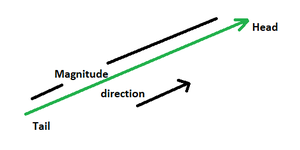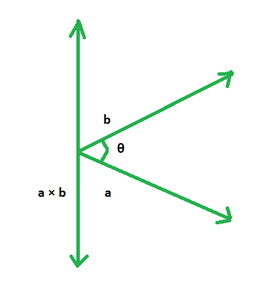# Cross Product of two Vectors

• Last Updated : 15 Feb, 2022

Vector is a two-dimensional entity with both magnitude and direction. A vector can be seen geometrically as a directed line segment with an arrow denoting the direction and a length equal to the magnitude of the vector. The vector’s direction is from the tail to the head. If two vectors have the same magnitude and direction, they are the same. This indicates that if we take a vector and translate it to a new point (without rotating it), the vector we get at the end is the same as the one we started with.### Cross Product of Two VectorsIn three-dimensional space, the cross product is a binary operation on two vectors. It generates a perpendicular vector to both the given vectors. a × b represents the vector product of two vectors, a and b. It produces a vector that is perpendicular to both a and b. Cross goods are another name for vector products. The outcome of the cross product of two vectors is a vector, which may be determined using the Right-hand Rule.

a × b = |a||b|sinθ

Cross Product Matrix

We can also derive the formula for the cross product of two vectors using the determinant of the matrix as given below.

A = ai + bj + ck

B = xi + yj + zk

A × B == (bz – cy)i + (cx – az)j + (ay – bx)k

### Sample Problems

Question 1. Find the cross product of the vectors:andSolution:

Given:Question 2. Find the cross product of the vectors:Solution:

Given:.Question 3. Find the cross product of the vectors:and.

Solution:

Given:and.Question 4. Find the cross product of the vectors:and.

Solution:

Given:.Question 5. Find the cross product of the vectors:and.

Solution:

Given:andQuestion 5. Find the cross product of the vectors:and.

Solution:

Given:and.Question 6. Find the cross product of the vectors:and.

Solution:

Given:and.Question 7. Find the cross product of the vectors:and.

Solution:

Given:and.My Personal Notes arrow_drop_up